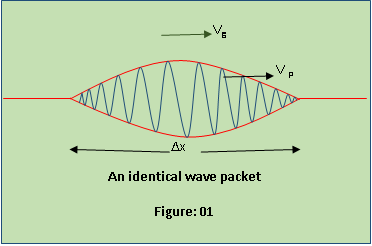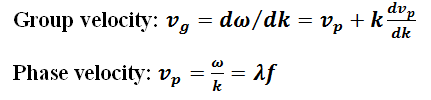# Difference Between Phase Velocity and Group Velocity

## Main Difference – Phase Velocity vs Group Velocity

According to quantum physics, particles sometimes behave like waves. In some cases, waves behave like particles. This property of particles and waves is known as the wave- particle duality. This dual nature of particles and waves allow us to represent a particle in terms of waves. Based on this concept, waves are used to represent particles as it is an essential step when we deal with particles in quantum physics. However, we must add many waves, having different angular frequencies and amplitudes in particular ways, in order to get a wave packet which represents a particle. Within this concept, there are two types of velocities namely phase velocity and group velocity. These two quantities have very different and particular definitions and properties. The main difference between Phase Velocity and Group Velocity is that phase velocity is greater than the group velocity in a normal medium. This article tries to differentiate those differences in detail.

## What is Group Velocity

In order to understand the nature of the group velocity, let’s consider a race. At any given instant, the velocity of a particular runner is different relative to others. Simply, the velocity of each individual runner within the group is different. But, they run within a group. The velocity at which the group of runners is moving can be considered as the group velocity in this simple example.In quantum physics, the idea of group velocity comes with the idea of wave packets or pulses. A wave packet can be obtained by adding up many waves having different wave numbers and amplitudes. So, the position of a particle can be localized within a specific region by using such a wave packet which represents a particle. The resultant amplitude of a wave packet differs with x as shown in fig 1. Nevertheless, this wave packet is a combination of many waves. The resultant wave packet represents the particle, and it is a group of many waves. So in this model, the velocity of the particle is equal to the velocity of the wave packet. In other words, the velocity of the particle is equal to the group of the waves with which the wave packet is made of. The velocity of the group or wave packet is called the group velocity. The group velocity can be mathematically expressed as, vg = Dω/dk. It is the velocity with which the particle moves.

Monochromatic harmonic waves cannot carry information through space. In order to convey information, superposition of many waves which gives a wave packet is needed.

##The phase velocity (wave velocity) of a wave is the velocity with which any given phase of the wave travels. For example, the point A of the wave (Fig: 02) travels with the phase velocity. It is given by  vp=ωk.

In a wave packet, the carrier wave travels with the phase velocity. But, when we deal with a particle or wave packet, the phase velocity isn’t important.

The relationship between the phase velocity and the group velocity depends on the material properties of the medium. In non-dispersive mediums, sv=vp. In normal dispersion mediums, svg <vp. But in anomalous mediums vg> vp.

In non- dispersive mediums, the phase velocity of waves does not depend on the wavelength. So, the phase velocity equals to the group velocity. For example, the phase velocity is equal to the group velocity when sound waves travel through the air as air is a non- dispersive medium for sound waves.

## Difference Between Phase Velocity and Group Velocity

### Velocity

Group velocity: In an anomalous medium, the group velocity is greater than the phase velocity.

Phase velocity: In a normal medium, the phase velocity is greater than the group velocity.

### Importance

Group velocity: The concept of group velocity is very important when a particle is represented by waves.

Phase velocity: The phase velocity is important when we deal with individual waves.

### In a wave packet

Group velocity: In a wave packet, the envelope travels with the group velocity.

Phase velocity: In a wave packet, the carrier wave travels with the phase velocity.

###